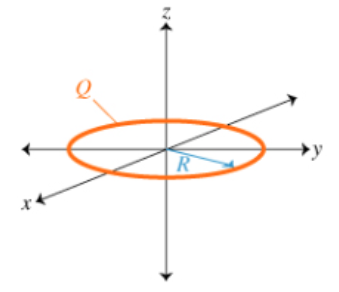# Problem: A ring with radius R and a uniformly distributed total charge Q lies in the xy plane, centered at the origin.Part A. What is the potential V(z) due to the ring on the z axis as a function of z? Express your answer in terms of Q, z, R, and ε0 or k = 1/(4πε0) Part B. What is the magnitude of the electric field E on the z axis as a function of z, for z &gt; 0? Express your answer in terms of some or all of the quantities Q, z, R, and ε0 or k = 1/(4πε0)

###### FREE Expert Solution

Electric potential:

$\overline{){\mathbf{V}}{\mathbf{=}}\frac{\mathbf{k}\mathbf{q}}{\mathbf{r}}}$

Part A

r = R = sqrt(R2 + z2)

85% (423 ratings)###### Problem Details

A ring with radius R and a uniformly distributed total charge Q lies in the xy plane, centered at the origin.Part A. What is the potential V(z) due to the ring on the z axis as a function of z? Express your answer in terms of Q, z, R, and ε0 or k = 1/(4πε0

Part B. What is the magnitude of the electric field E on the z axis as a function of z, for z > 0? Express your answer in terms of some or all of the quantities Q, z, R, and ε0 or k = 1/(4πε0)